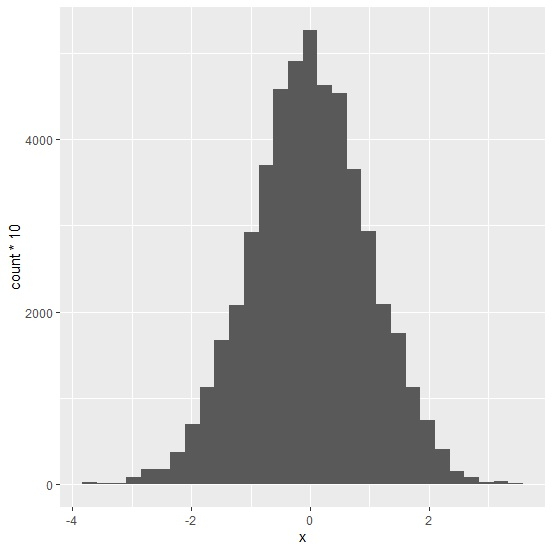# How to create a histogram with Y-axis values as count using ggplot2 in R?

To create a histogram with Y-axis values as count using ggplot2 in R, we can follow the below steps −

• First of all, create a data frame.
• Then, use geom_histogram function of ggplot2 package with aes to create the histogram with Y-axis values as count.

## Create the data frame

Let's create a data frame as shown below −

Live Demo

> df<-data.frame(x=rnorm(5000))
> head(df,20)

On executing, the above script generates the below output(this output will vary on your system due to randomization) −

          x
1    -0.008015477
2    -0.981227322
3     1.144050354
4     0.207177231
5     0.179782914
6     0.380085361
7    -0.828305873
8    -0.909047732
9     1.946699123
10    0.384881892
11   -1.107651249
12   -1.290435936
13   -0.305554288
14   -0.427279557
15   -0.587864723
16   -0.295237215
17    0.178995066
18   0.476328701
19   -0.392095095
20   -1.215651876

## Create a histogram with Y-axis values as count using ggplot2

Using geom_histogram function of ggplot2 package to create the histogram with Y-axis values as count for the data in data frame df −

> df<-data.frame(x=rnorm(5000))
> library(ggplot2)
> ggplot(df,aes(x))+geom_histogram(aes(y=..count..*10),bins=30)

## Output